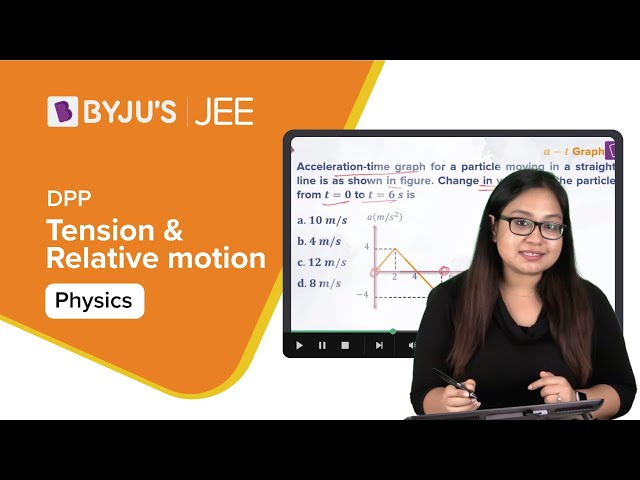Checkout JEE MAINS 2022 Question Paper Analysis : Checkout JEE MAINS 2022 Question Paper Analysis :

# Dimensions of Tension

## Dimensional Formula of Tension

The dimensional formula of tension is given by,

M1 LT-2

Where,

• M = Mass
• L = Length
• T = Time

### Derivation

Since tension is a type of force, therefore, the dimensions of Tension = dimensions of Force

⇒ Force is given by, F = M × a . . . (1)

The dimensions of,

Mass = [ML0 T0] . . . . (2)

Acceleration = [MLT-2] . . . . (3)

On substituting equation (2) and (3) in equation (1) we get,

Force = M × a

Or, Tension = [ML0 T0] × [M0 LT-2] = M1 LT-2.

Therefore, the tension is dimensionally represented as M1 LT-2.

### Tension and Relative Motion – Video Lesson